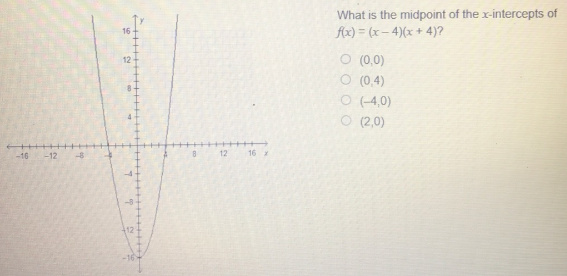# Midpoint of the x intercepts of f(x)=(x-4)(x+4)

Midpoint of the x intercepts of f(x)=(x-4)(x+4)